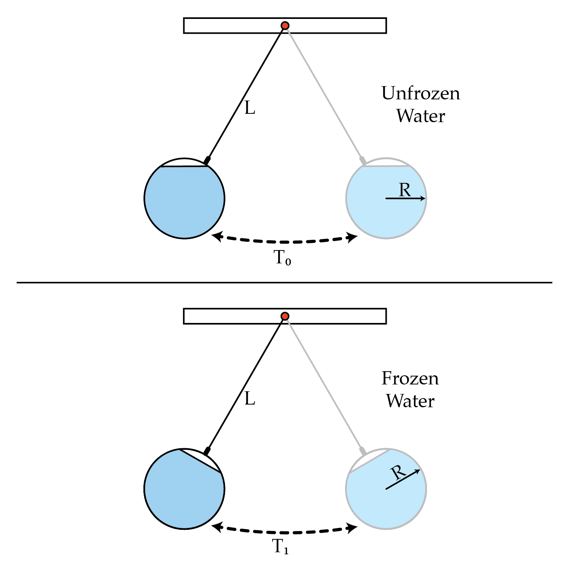# A frozen pendulum

A pendulum is constructed as a light thin-walled sphere filled up with water and suspended from the ceiling by a rigid rod of negligible mass. The radius of the spherical container is R and the distance between the pivot point and the center of mass of the system is L. The period of small oscillations is $T_{0}$. Due to a temperature drop in the room the water froze and the pendulum began to oscillate with a different frequency, corresponding to the period $T_{1}$. Determine $\frac{T_{1}}{T_{0}}$ if $\frac{L}{R}=2$. Neglect the viscosity (friction) of the water and the change of its volume after freezing.×